# RD Sharma Class 8 Solutions Chapter 4 Cubes and Cube Roots Ex 4.4

In this chapter, we provide RD Sharma Class 8 Solutions Chapter 4 Cubes and Cube Roots Ex 4.4 for English medium students, Which will very helpful for every student in their exams. Students can download the latest RD Sharma Class 8 Solutions Chapter 4 Cubes and Cube Roots Ex 4.4 Maths pdf, free RD Sharma Class 8 Solutions Chapter 4 Cubes and Cube Roots Ex 4.4 Maths book pdf download. Now you will get step by step solution to each question.

### RD Sharma Solutions for Class 8 Chapter 4 Cubes and Cube Roots Ex 4.4Download PDF

Question 1.
Find the cube roots of each of the following integers :
(i) -125
(ii) -5832
(iii) -2744000
(iv) -753571
Solution: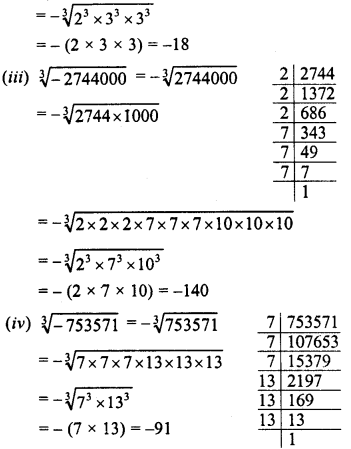Question 2.
Show that :Solution: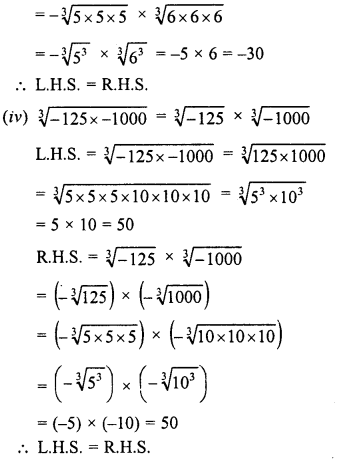Question 3.
Find the cube root of each of the following numbers :
(i) 8 x 125
(ii) -1728 x 216
(iii) -27 x 2744
(iv) -729 x -15625
Solution: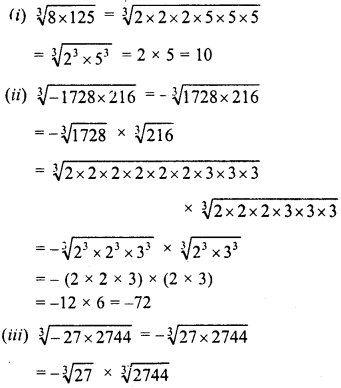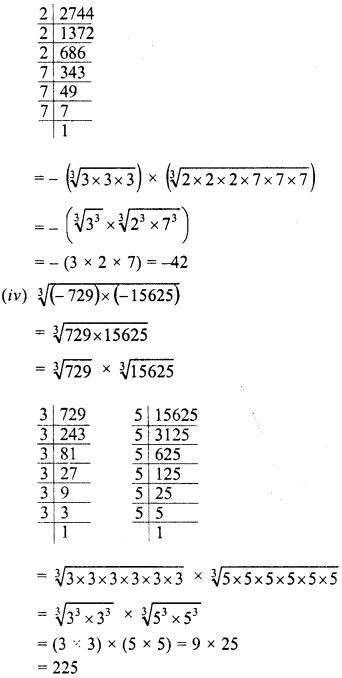Question 4.
Evaluate :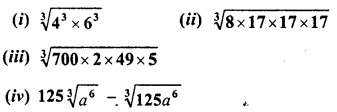Solution: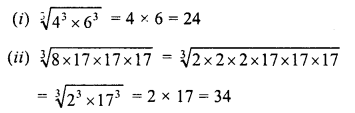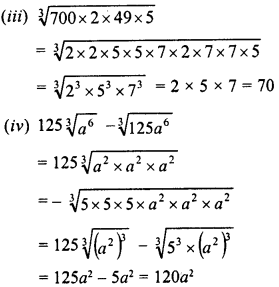Question 5.
Find the cube root of each of the following rational numbers.Solution: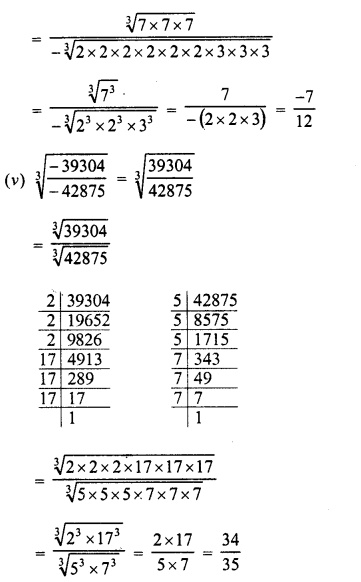Question 6.
Find the cube root of each of the following rational numbers :
(i) 0.001728
(ii) 0.003375
(iii) 0.001
(iv) 1.331
Solution:Question 7.
Evaluate each of the following :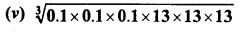Solution: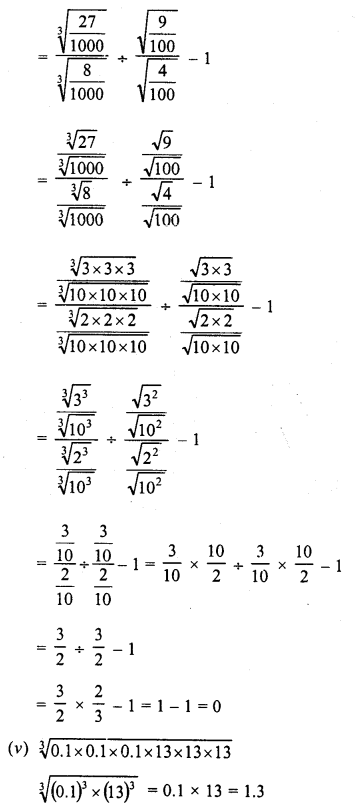Question 8.
Show that :Solution: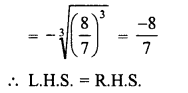Question 9.
Fill in the Blanks :Solution:Question 10.
The volume of a cubical box is 474.552 cubic metres. Find the length of each side of the box.
Solution:Question 11.
Three numbers are to one another 2:3: 4. The sum of their cubes is 0.334125. Find the numbers.
Solution:Question 12.
Find side of a cube whose volume is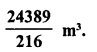Solution: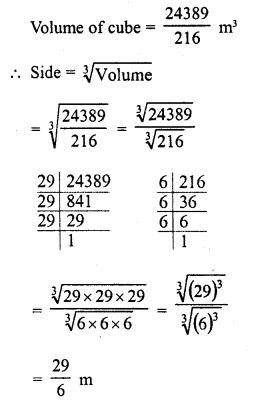Question 13.
Evaluate :Solution: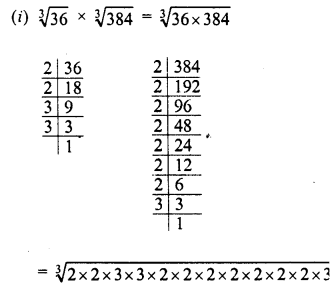Question 14.
Find the cube root of the numbers : 2460375,20346417,210644875,57066625 using the fact that
(i) 2460375 = 3375 x 729
(i) 20346417 = 9261 x 2197
(iii) 210644875 = 42875 x 4913
(iv) 57066625 = 166375 x 343
Solution: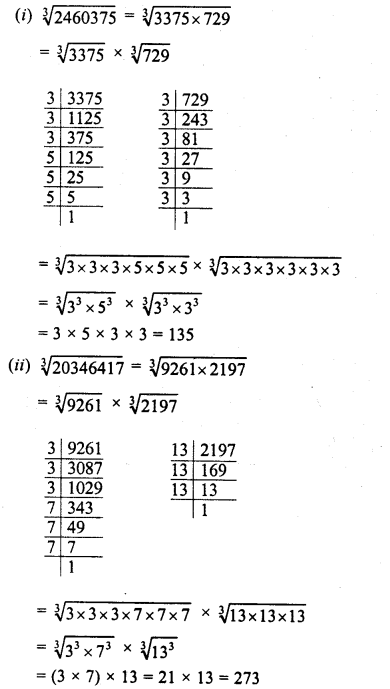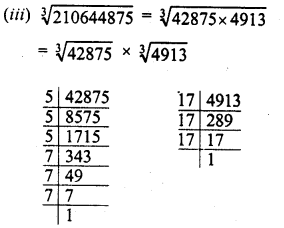Question 15.
Find the units digit of the cube root of the following numbers ?

(i) 226981
(ii) 13824
(iii) 571787
(iv) 175616
Solution:
(i)
226981
In it unit digit is 1
∴The units digit of its cube root will be = 1
(∵ 1 x 1 x 1 = 1)
∴Tens digit of the cube root will be = 6
(ii) 13824
∵ The units digit of 13824 = 4
(∵ 4 X 4 X 4 = 64)
∴Units digit of the cube root of it = 4
(iii) 571787
∵ The units digit of 571787 is 7
∴The units digit of its cube root = 3
(∵ 3 x 3 x 3 = 27)
(iv) 175616
∵ The units digit of 175616 is 6
∴The units digit of its cube root = 6
(∵ 6 x 6 x 6=216)

Question 16.
Find the tens digit of the cube root of each of the numbers in Question No. 15.

Solution:
(i) In 226981
∵ Units digit is 1
∴Units digit of its cube root = 1
We have 226
(Leaving three digits number 981)
63 = 216 and 73 = 343
∴63 ∠226 ∠ T
∴The ten’s digit of cube root will be 6
(ii) In 13824
Leaving three digits number 824, we have 13
∵ (2)3 = 8, (3)3 = 27
∴23 ∠13 ∠3′
∴Tens digit of cube root will be 2
(iii) In 571787
Leaving three digits number 787, we have 571
83 = 512, 93 = 729
∴ 83 ∠571 ∠93
Tens digit of the cube root will be = 8
(iv) In 175616
Leaving three digit number 616, we have 175
∵ 53 = 125, 63 = 216
∴53 ∠175 ∠63
∴Tens digit of the cube root will be = 5

All Chapter RD Sharma Solutions For Class 8 Maths

—————————————————————————–

All Subject NCERT Exemplar Problems Solutions For Class 8

All Subject NCERT Solutions For Class 8

*************************************************

I think you got complete solutions for this chapter. If You have any queries regarding this chapter, please comment on the below section our subject teacher will answer you. We tried our best to give complete solutions so you got good marks in your exam.

If these solutions have helped you, you can also share rdsharmasolutions.in to your friends.